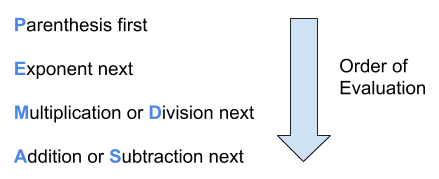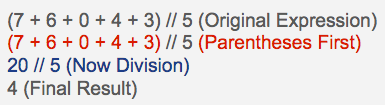# Order of Operations in Python

Now we understand expressions and how to use Python as a calculator

Let's understand a very important concept - order of operations. Let's calculate the average of five numbers `7, 6, 0, 4, and 3`Apply the above formula to calculate the average. Divide sum of numbers `7 + 6 + 0 + 4 + 3` by count of numbers `5`

``````7 + 6 + 0 + 4 + 3 // 5
``````

Execute the above expression in the Jupyter notebook and check the average. It prints the average as `17`. Is average correct?

No, it is wrong. `7 + 6 + 0 + 4 + 3` is `20` and the count of numbers is `5`. So average should be `4`

So what is wrong and how do find the correct average?

Hope you remember `PEMDAS` from your High School math classes. `PEMDAS` defines the order of operations.

Below is the order of operationsNotice `Multiplication or Division` and `Addition or Subtraction` are on the same level. We can either evaluate multiplication or division whichever comes first. Similarity we can either evaluate addition or subtraction whichever comes first.

Now since we know the order of operations, we can figure out why the above average calculation was wrong. Our code first evaluated `3 // 5` and got the result as `0` and then it added `7, 6, 0, 4 and 0` which resulted in `17`. Below is the explanation of why we got the incorrect averageWhat is the correct way to calculate average then? Answer - Wrap the first expression in parenthesis.

``````(7 + 6 + 0 + 4 + 3) // 5
``````

Below is the explanation of why above expression gives the correct averageINSTRUCTIONS

Now we know the order of operations. Let's write an expression to calculate the simple interest.

``````Simple Interest = ( Principal * Rate * Time ) / 100
``````

Question - Sam deposited \$5, 000 in a bank having 10% annual simple interest rate. How much interest will Sam earn in 4 years?

• Here, the principal is `5000`
• The rate of interest is `10`
• The time is `4`
• Plug in values in the simple interest formula and compute the interest
• Fill in the integer value of computed interest below

The simple interest is

No hints are availble for this assesment

Answer is not availble for this assesment

Note - Having trouble with the assessment engine? Follow the steps listed here